## Saturday, February 27, 2016

### Cardinality Constrained Portfolio Optimization: MIQP without MIQP Solver

The optimization toolbox of Matlab does not contain an MIQP (Mixed Integer Quadratic Programming) solver. So when they discuss a cardinality constrained portfolio problem (this means only a certain number of assets can be in the portfolio), an interesting cutting plane technique is used instead. This method will solve a series of linear MIP models instead of attacking the MIQP model directly.

Let’s first formulate the problem as proper MIQP model. First here is how the data is prepared:

##### Data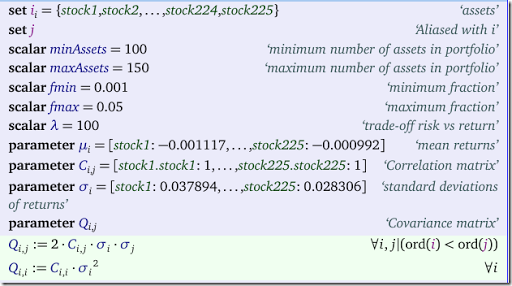The main deviation from the original model is that we don’t store the variance-covariance matrix $$Q$$ as a full dense matrix but as an upper-triangular matrix (it includes the diagonal). We multiply the off-diagonal elements by two, so that the expression $$\sum_{i,j} x_i Q_{i,j} x_j$$ will yield exactly the same result for any $$x$$. Basically we replace expressions like $$x_1 Q_{1,2} x_2 +x_2 Q_{2,1} x_1$$ by $$2 x_1 Q_{1,2} x_2$$. The sole purpose of this trick is to have fewer non-zero elements in the quadratic form $$x^TQx$$. This really can help for larger problems (this looks mainly a GAMS problem: GAMS is slower in generating the model when the nonzero count of the Q matrix increases; it does not make much difference for the QP solver).

The model itself looks like:

##### MIQP Model##### Modeling trick

In the original model the variable $$K$$ was not present and the min- and max-fraction constraints were formulated as:

 \begin{align} &\sum_i \delta_i \ge \text{minAssets}\\ &\sum_i \delta_i \le \text{maxAssets} \end{align}
This duplicates a summation. Our formulation adds a single variable (with a lower- and upper-bound) but we get rid of an equation that includes a summation. As a result we reduce the number of non-zero elements in the constraint matrix.
##### Cutting Plane Algorithm

In the cutting plane method we replace the quadratic objective

 $\min\> \lambda \sum_{i,j} x_i Q_{i,j} x_j - \sum_i \mu_i x_i$
by
 \begin{align} \min\>& \lambda y - \sum_i \mu_i x_i\\ & y \ge - {x^*_k}^T Q x^*_k + 2{x^*_k}^T Q x \> \text{for $$k=1,...,K-1$$} \end{align}
where $$K$$ is the current iteration or cycle, and $$x^*_k$$ is the optimal MIP solution from the k-th iteration. This is essentially a Taylor approximation.

Here is our version of the algorithm: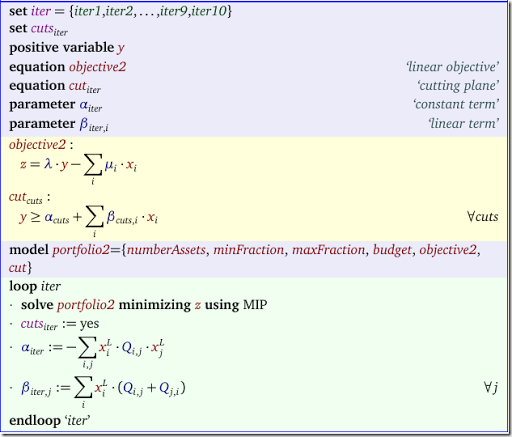In this second model we replaced the original objective by the new linear objective and the cuts. The equation cut is indexed by a dynamic set cuts that grows during the iteration loop. We start the iteration scheme with an empty set cuts. Note that in the calculation of $$\beta$$ we changed the expression from $$2{x^*_k}^T Q$$ to $${x^*_k}^T (Q+Q^T)$$. This is to compensate for the upper-triangularization trick we used before.

The results are:

miqp    0.036039
iter1  -0.002430
iter2   0.013922
iter3   0.025362
iter4   0.034862
iter5   0.035138
iter6   0.035646
iter7   0.035731
iter8   0.035776
iter9   0.035790
iter10  0.035828

We see the objectives converge towards the optimal MIQP objective.

##### References
1. Mathworks, Mixed-Integer Quadratic Programming Portfolio Optimization.
2. J. E. Kelley, Jr. "The Cutting-Plane Method for Solving Convex Programs." J. Soc. Indust. Appl. Math. Vol. 8, No. 4, pp. 703-712, December, 1960.

## Friday, February 26, 2016

##### R

The R package quadprog contains a QP (Quadratic Programming) algorithm that solves the following problem:

 \begin{align} \min\>&\frac{1}{2}x^TQx-d^Tx \\ &A_1^Tx = b_1 \\ &A_2^Tx \ge b_2 \end{align}

The funny factor $$\frac{1}{2}$$ is often used in QP algorithms. I suspect this is largely for historical reasons (the background may be that the gradients are then just the $$q_{i,j}$$ values).  Notice in the input that the first $$m_1$$ constraints are equality constraints and the next $$m_2$$ constraints are $$\ge$$ inequalities. Bounds need to be represented as constraints and $$\le$$ constraints $$a^Tx \le b$$ need to be passed on as $$-a^Tx \ge -b$$. The fact that linear equations are expressed as $$A^Tx = b$$ is unusual ($$Ax = b$$ is far more conventional).

Here is a small example on how one can use it to solve a small portfolio problem:

 \begin{align} \min\>&\frac{1}{2}x^TQx-\lambda d^Tx \\ & \sum_i x_i=1 \\ & 0 \le x_i \le 0.8 \end{align}

where we assume $$\lambda=1$$.

The R code can look like:

> library(quadprog)
> Q <- rbind(c(0.00020817,0.00016281,0.00009747),
+           c(0.00016281,0.00026680,0.00009912),
+           c(0.00009747,0.00009912,0.00019958))
> Q
[,1]       [,2]       [,3]
[1,] 0.00020817 0.00016281 0.00009747
[2,] 0.00016281 0.00026680 0.00009912
[3,] 0.00009747 0.00009912 0.00019958
> d = c(0.1,0.05,0.1)
> d
 0.10 0.05 0.10
> A = rbind(c(1,1,0,0,-1,0,0),
+           c(1,0,1,0,0,-1,0),
+           c(1,0,0,1,0,0,-1))
> A
[,1] [,2] [,3] [,4] [,5] [,6] [,7]
[1,]    1    1    0    0   -1    0    0
[2,]    1    0    1    0    0   -1    0
[3,]    1    0    0    1    0    0   -1
> b = c(1,0,0,0,-0.8,-0.8,-0.8)
> b
  1.0  0.0  0.0  0.0 -0.8 -0.8 -0.8
> solve.QP(Q,d,A,b,1)
$solution  0.4798177 0.0000000 0.5201823$value
 -0.09992471

$unconstrained.solution  507.7568 -265.4413 384.9057$iterations
 6 3

$Lagrangian  0.09984941 0.00000000 0.04997909 0.00000000 0.00000000 0.00000000 0.00000000$iact
 1 3
##### Javascript

Here we try the javascript version of this algorithm, found here: www.numericjs.com.  We throw the same data at it. We use the notebook-like interface to enter the data and solve the problem: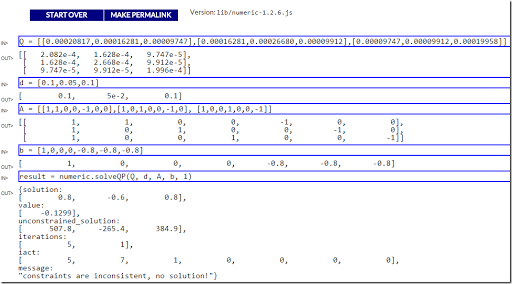Looks like the constraints cause some problems here. The unconstrained solution is the same. But when the constraints are added to the problem the algorithm fails miserably. At least on this problem, R seems to be a winner.

##### Matlab

Matlab also contains a QP algorithm called quadprog, documented here. The usage is a little bit more natural in my opinion: they don’t transpose A and they allow lower- and upper-bounds to be specified directly.

##### References

D. Goldfarb and A. Idnani (1982). Dual and Primal-Dual Methods for Solving Strictly Convex Quadratic Programs. In J. P. Hennart (ed.), Numerical Analysis, Springer-Verlag, Berlin, pages 226–239.

D. Goldfarb and A. Idnani (1983). A numerically stable dual method for solving strictly convex quadratic programs. Mathematical Programming, 27, 1–33

## Tuesday, February 23, 2016

### Self-descriptive numbers: Prolog vs. MIP

In http://stackoverflow.com/questions/35485185/puzzle-taken-from-gardner a small problem is given: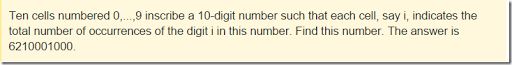I.e. there are 6 zeroes in the number, 2 ones, 1 two, and 1 six. This is called a self-descriptive number. The post is talking about a Prolog solution. Of course we can use a MIP solver instead of Prolog: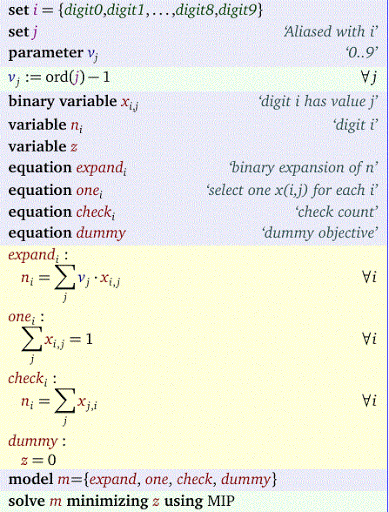The solution looks ok:

---- VAR n  digit i

LOWER          LEVEL          UPPER         MARGINAL

digit0        -INF            6.0000        +INF             .
digit1        -INF            2.0000        +INF             .
digit2        -INF            1.0000        +INF             .
digit3        -INF             .            +INF             .
digit4        -INF             .            +INF             .
digit5        -INF             .            +INF             .
digit6        -INF            1.0000        +INF             .
digit7        -INF             .            +INF             .
digit8        -INF             .            +INF             .
digit9        -INF             .            +INF             .

The very short Prolog code as mentioned in the post is:

:- use_module(library(clpfd)).
ten_cells(Ls) :-
numlist(0, 9, Nums),
pairs_keys_values(Pairs, Nums, Ls),
global_cardinality(Ls, Pairs).

It is extremely short but not exactly very readable or intuitive (I guess that was not one of the design criteria for Prolog – see here for a discussion about write-only languages with a particularly illuminating APL example showcasing this concept). I prefer here the mathematical optimization model. A plus for the Prolog version is that it also verifies there is only a single solution for n=10.

### R: lazy load DB files

R can read data very fast and conveniently from .Rdata files. E.g.

> load("indus89.rdata")
> length(ls())
 275

For this data set we have a lot of data: 275 objects are loaded: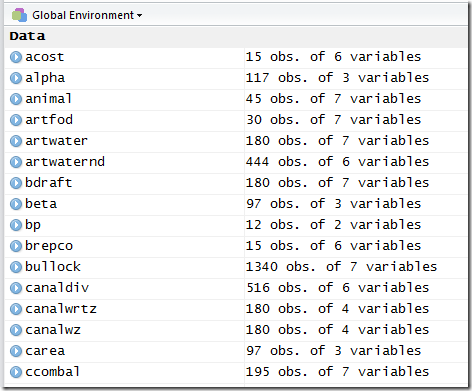If we just want to inspect a few of these objects, it may be more convenient to use a lazy load DB format. Such a database consists of two files: an .RDB file with data and an .RDX file with an index indicating where each object is located in the .RDB data file. The .RDB data file is slightly larger than the corresponding .Rdata file: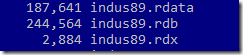This is because each object is stored and compressed individually.

> lazyLoad("indus89")
NULL
> length(ls())
 275

This will only load the index of the data. The RStudio environment shows: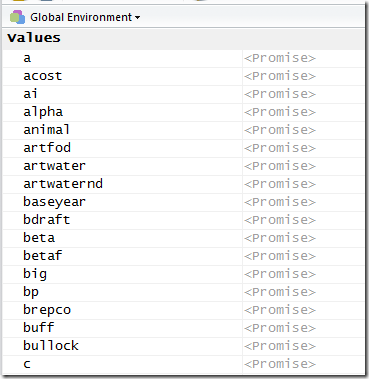Now, as soon as we do anything with the data, it will load it. This is the “lazy” loading concept. E.g. lets just print alpha:

> head(alpha)
cq   z1 value
1 basmati nwfp     6
2 basmati  pmw     6
3 basmati  pcw    21
4 basmati  psw    21
5 basmati  prw    21
6 basmati scwn     6

Now suddenly the Rstudio environment shows: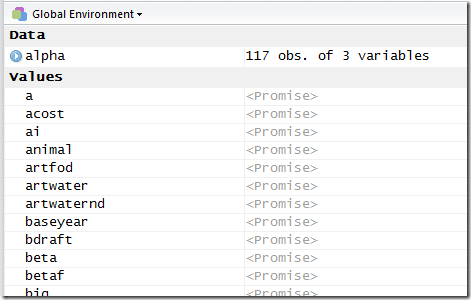We can also lazy load a subset of the objects. E.g. if we want to load all symbols starting with the letter ‘a’ we can do:

> lazyLoad("indus89",filter=function(s){grepl("^a",s)})
NULL
> length(ls())
 8

All symbols containing ‘water’ can be loaded as follows:

> lazyLoad("indus89",filter=function(s){grepl("water",s)})
NULL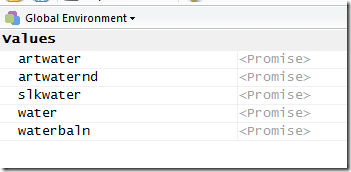This format seems an interesting alternative to store larger data sets, allowing more selective and lazy loading.

##### Update

As indicated in the comments below, the lazyLoad function is described as being for internal use. So usage may require a certain braveness. I am sure  renaming the function to myLazyLoad does not count as a solution for this.

Alternatives mentioned in the comments (of course if the venerable Bill Venables makes a comment I better pay attention):

## Sunday, February 21, 2016

### xor as linear inequalities

I came across a question about using an xor condition in a MIP model. Here is a summary of the answer.

Expressing $$z=x\cdot y$$ (or $$z=x\text{ and }y$$ ) where $$x,y,z \in \{0,1\}$$ as a set of linear constraints is well-known:

and:     \begin{align} &z \le x \\ &z \le y \\ &z \ge x+y-1 \end{align} $$\>\>\>\Longleftrightarrow\>\>\>$$
 $$z$$ $$x$$ $$y$$ $$0$$ $$0$$ $$0$$ $$0$$ $$0$$ $$1$$ $$0$$ $$1$$ $$0$$ $$1$$ $$1$$ $$1$$
The relation $$z=x\text{ xor }y$$ is slightly more complicated. The xor (exclusive-or) condition can also be written as $$z = x<>y$$, i.e. $$z$$ is 1 if $$x$$ and $$y$$ are different (and $$z$$ is zero if they are the same). This we can write as the following set of linear inequalities:
xor:     \begin{align} & z \le x+y\\ & z \ge x-y \\ & z \ge y-x \\ & z \le 2 -x - y \end{align} $$\>\>\>\Longleftrightarrow\>\>\>$$
 $$z$$ $$x$$ $$y$$ $$0$$ $$0$$ $$0$$ $$1$$ $$0$$ $$1$$ $$1$$ $$1$$ $$0$$ $$0$$ $$1$$ $$1$$
To be complete $$z=x\text{ or }y$$ can be written as:
or:     \begin{align} & z \le x+y\\ & z \ge x \\ & z \ge y \end{align} $$\>\>\>\Longleftrightarrow\>\>\>$$
 $$z$$ $$x$$ $$y$$ $$0$$ $$0$$ $$0$$ $$1$$ $$0$$ $$1$$ $$1$$ $$1$$ $$0$$ $$1$$ $$1$$ $$1$$
In all of this we assumed $$x,y$$ and $$z$$ are binary variables.

## Wednesday, February 17, 2016

### Strange restriction

In this post the question is posed how to model the restriction
$x \in \{1,2,3,4,5,6,8,10,12,..,100\}.$
Note that this is $$\{1,2,3,4,5\} \cup \{6,8,10,..,98,100\}$$.  Never seen a thing like this, but here is a way to model this in a MIP model:
\begin{align} & 6 - (1-\delta)M \le x \le 5 + \delta M\\ & 2y -(1-\delta)M \le x \le 2y+(1-\delta)M \\ & 1 \le x \le 100 \\ & x \> \text{integer}\\ & y \> \text{integer}\\ & \delta \> \text{binary} \end{align}
Note that this essentially means:
\begin{align} & \delta=0 \implies x\le 5 \\ & \delta=1 \implies x\ge 6, x = 2y \end{align}
We can use $$M=100$$ (or even refine that a little bit).

## Tuesday, February 16, 2016

### Two nonlinear formulations

##### Problem Description

I am not at all sure the physics make sense, but let’s focus on the mathematics of this model.

##### Formulation A

An MINLP formulation could be:The idea is:

• If $$y_i=0$$ (oven is turned off) we force $$x_i=0$$ (equation Off). Also we have $$loss_i=0$$ as we multiplied the loss function by $$y_i$$.
• If $$y_i=1$$ (oven is turned on), we let the solver decide on $$x_i$$. In almost all cases it will choose a value > 0 as it wants to be somewhat close to $$a_i$$. But if $$x_i$$ would become zero something special happens: suddenly $$y_i=0$$ becomes a better solution.
• We don’t have to model explicitly $$x_i=0 \implies y_i=0$$. If $$x_i=0$$ then the objective would have a preference for $$y_i=0$$ as this will improve the objective.

This model is no longer quadratic. For small instances like this we can use a global solver without a problem.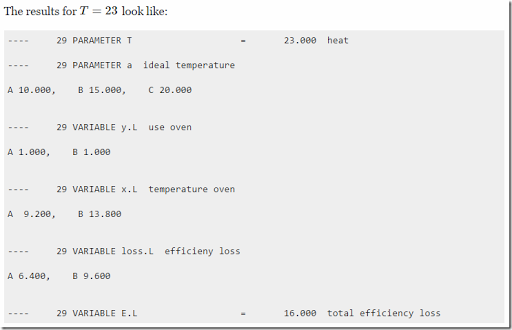##### Formulation B

If we can make the problem quadratic and convex we can solve the problem with many more solvers. So here is a formulation that does just that:Here we have:

• If $$y_i=0$$ (oven is turned off) we force $$x_i=0$$ (equation Off).  The slack $$s_i$$ is left floating. This will cause the algorithm to choose a slack such that the loss is zero.
• If $$y_i=1$$ (oven is turned on) we force $$s_i=0$$ (equations On1,On2).

As this model is convex all MIQP solvers can handle this.

##### Conic formulation

For a compact conic formulations of this problem see: http://blog.mosek.com/2016/03/reformulating-non-convex-minlp-as-misocp.html

When using load(), R will load all objects in the “global environment”, i.e. the interactive workspace:

> load("results.rdata",verbose=T)
i
f
c
x

If you want to load data but not clutter the global environment you can use some esoteric constructs:

> e = local({load("results.rdata", verbose=T); environment()})
i
f
c
x

Here we load all data into a new environment e.  As suggested by Paul Rubin in the comments, a more readable version of this is:

> e <- new.env()          # by default it is a child of the parent environment
i
f
c
x

To interact with the data you can do things like:

> ls(e)
 "c" "f" "i" "x"
> e$c i j value 1 seattle new-york 0.225 2 seattle chicago 0.153 3 seattle topeka 0.162 4 san-diego new-york 0.225 5 san-diego chicago 0.162 6 san-diego topeka 0.126 The$ operator can be used to access the data and ls() is a way to list what is inside the environment. There is an additional function ls.str() to show all structures.

> ls.str(e)
c : 'data.frame':	6 obs. of  3 variables:
$i : chr "seattle" "seattle" "seattle" "san-diego" ...$ j    : chr  "new-york" "chicago" "topeka" "new-york" ...
$value: num 0.225 0.153 0.162 0.225 0.162 0.126 f : num 90 i : chr [1:2] "seattle" "san-diego" x : 'data.frame': 6 obs. of 6 variables:$ i       : chr  "seattle" "seattle" "seattle" "san-diego" ...
$j : chr "new-york" "chicago" "topeka" "new-york" ...$ level   : num  50 300 0 275 0 275
$marginal: num 0 0 0.036 0 0.009 ...$ lower   : num  0 0 0 0 0 0
$upper : num Inf Inf Inf Inf Inf ... Less typing is needed after an attach such that environment e is added to the search path: > attach(e) > c i j value 1 seattle new-york 0.225 2 seattle chicago 0.153 3 seattle topeka 0.162 4 san-diego new-york 0.225 5 san-diego chicago 0.162 6 san-diego topeka 0.126 Use detach() to remove it from the search path again. With rm() we can delete objects inside an environment (or a complete environment). > rm(c,envir=e) > rm(e) More information: http://adv-r.had.co.nz/Environments.html. ## Friday, February 12, 2016 ### Move GAMS data into R: gdxrrw vs gdx2r #### GAMS tool GDXRRW ##### Script:$set script script.R
$set rpath C:\Program Files\R\R-3.2.3\bin\x64\Rscript set i /i1*i200/ ; alias (i,j,k); parameter p(i,j,k); p(i,j,k) = uniform(0,1); execute_unload "p" ,p;$onecho > %script%
library(gdxrrw)

p<-rgdx.param("p.gdx","p")
$offecho execute '="%rpath%" "%system.fp%%script%"'; ##### Log: --- Job Untitled_5.gms Start 02/12/16 18:26:13 24.6.1 r55820 WEX-WEI x86 64bit/MS Windows GAMS 24.6.1 Copyright (C) 1987-2016 GAMS Development. All rights reserved Licensee: Erwin Kalvelagen G150803/0001CV-GEN Amsterdam Optimization Modeling Group DC10455 --- Starting compilation --- Untitled_5.gms(17) 3 Mb --- Starting execution: elapsed 0:00:00.007 --- Untitled_5.gms(8) 258 Mb 3 secs --- Untitled_5.gms(9) 261 Mb --- GDX File C:\tmp\p.gdx --- Untitled_5.gms(17) 261 Mb i j k p 1 i1 i1 i1 0.1717471 2 i1 i1 i2 0.8432667 3 i1 i1 i3 0.5503754 4 i1 i1 i4 0.3011379 5 i1 i1 i5 0.2922121 6 i1 i1 i6 0.2240529 *** Status: Normal completion --- Job Untitled_5.gms Stop 02/12/16 18:26:41 elapsed 0:00:27.719 #### New tool gdx2r ##### Script:$set script script.R
$set rpath C:\Program Files\R\R-3.2.3\bin\x64\Rscript set i /i1*i200/ ; alias (i,j,k); parameter p(i,j,k); p(i,j,k) = uniform(0,1); execute_unload "p" ,p;$onecho > %script%

$offecho execute '=gdx2r -i p.gdx -o p.rdata -compression no' ; execute '="%rpath%" "%system.fp%%script%"' ; ##### Log: --- Job Untitled_7.gms Start 02/12/16 18:35:14 24.6.1 r55820 WEX-WEI x86 64bit/MS Windows GAMS 24.6.1 Copyright (C) 1987-2016 GAMS Development. All rights reserved Licensee: Erwin Kalvelagen G150803/0001CV-GEN Amsterdam Optimization Modeling Group DC10455 --- Starting compilation --- Untitled_7.gms(18) 3 Mb --- Starting execution: elapsed 0:00:00.007 --- Untitled_7.gms(9) 261 Mb --- GDX File C:\tmp\p.gdx --- Untitled_7.gms(17) 261 Mb GDX2R v 0.1 Copyright (c) 2016 Amsterdam Optimization Modeling Group LLC 64 bit version Input file:p.gdx Output file:p.rdata File format:Uncompressed Buffer size:4096 Strings as factors:True Uels:200 (unique strings in input data) Symbols:1 Exporting symbols: p (Converting 8000000 records from a 3 dimensional parameter to a data frame) Time:3.75 seconds --- Untitled_7.gms(18) 261 Mb i j k value 1 i1 i1 i1 0.1717471 2 i1 i1 i2 0.8432667 3 i1 i1 i3 0.5503754 4 i1 i1 i4 0.3011379 5 i1 i1 i5 0.2922121 6 i1 i1 i6 0.2240529 *** Status: Normal completion --- Job Untitled_7.gms Stop 02/12/16 18:35:23 elapsed 0:00:09.487 #### Discussion It is somewhat surprising that gdx2r is so much faster on this task. Here are the steps in the first GDXRRW script: 1. Generate data and write GDX file 2. Start R 3. Read GDX file The gdx2r script does actually much more I/O: 1. Generate data and wite GDX file 2. Read GDX file 3. Write .Rdata file 4. Start R 5. Read .Rdata file Looking at these steps I would expect the first GDXRRW script to be twice as fast as the second gdx2r script while actually it is three times as slow (so we are a factor six off). ## Thursday, February 11, 2016 ### R: Factors vs Strings on large data sets When exporting data sets in R’s .Rdata format, one of the things to consider is how string vectors are exported. I can now write factors in my .Rdata writer, so we can do some experiments on exporting a string column just as strings or as a factor.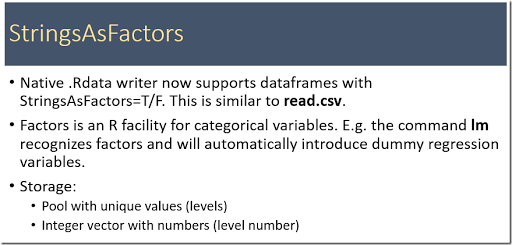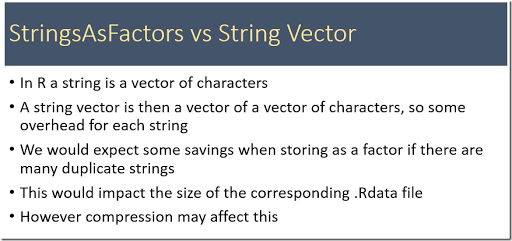Here is an illustration how things are stored in an .Rdata file: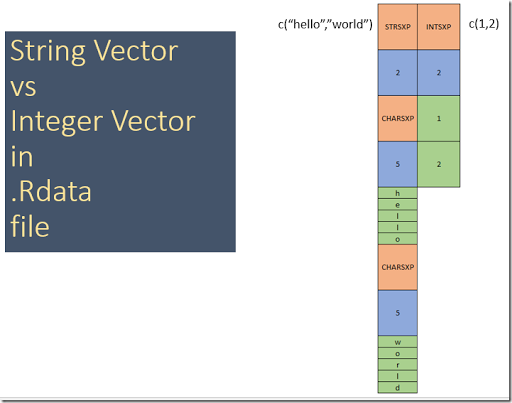There is some overhead with each string: 8 bytes. This can add up. An integer vector takes much less space. When we generate a large dataframe using gdx2r we see the following.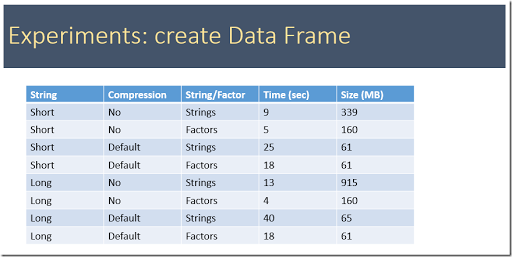The “short” data sets are as follows. For the “short” data set: set i /i1*i200/; alias (i,j,k); parameter p(i,j,k); p(i,j,k) = uniform(0,1); which exported to an .Rdata file (with StringsAsFactors=F) imported in R will look like: > load("p.rdata") > head(p) i j k value 1 i1 i1 i1 0.1717471 2 i1 i1 i2 0.8432667 3 i1 i1 i3 0.5503754 4 i1 i1 i4 0.3011379 5 i1 i1 i5 0.2922121 6 i1 i1 i6 0.2240529 > str(p) 'data.frame': 8000000 obs. of 4 variables:$ i    : chr  "i1" "i1" "i1" "i1" ...
$j : chr "i1" "i1" "i1" "i1" ...$ k    : chr  "i1" "i2" "i3" "i4" ...
$value: num 0.172 0.843 0.55 0.301 0.292 ... The “large” data sets look like: set i /amuchlongernamefortesting1*amuchlongernamefortesting200/; alias (i,j,k); parameter p(i,j,k); p(i,j,k) = uniform(0,1); Here we export to an .Rdata file with StringsAsFactors=T: > load("p2.rdata") > head(p) i j k value 1 amuchlongernamefortesting1 amuchlongernamefortesting1 amuchlongernamefortesting1 0.1717471 2 amuchlongernamefortesting1 amuchlongernamefortesting1 amuchlongernamefortesting2 0.8432667 3 amuchlongernamefortesting1 amuchlongernamefortesting1 amuchlongernamefortesting3 0.5503754 4 amuchlongernamefortesting1 amuchlongernamefortesting1 amuchlongernamefortesting4 0.3011379 5 amuchlongernamefortesting1 amuchlongernamefortesting1 amuchlongernamefortesting5 0.2922121 6 amuchlongernamefortesting1 amuchlongernamefortesting1 amuchlongernamefortesting6 0.2240529 > str(p) 'data.frame': 8000000 obs. of 4 variables:$ i    : Factor w/ 200 levels "amuchlongernamefortesting1",..: 1 1 1 1 1 1 1 1 1 1 ...
$j : Factor w/ 200 levels "amuchlongernamefortesting1",..: 1 1 1 1 1 1 1 1 1 1 ...$ k    : Factor w/ 200 levels "amuchlongernamefortesting1",..: 1 2 3 4 5 6 7 8 9 10 ...
\$ value: num  0.172 0.843 0.55 0.301 0.292 ...

The timings are indeed what we expected:

• If not compressed then the .Rdata files are much smaller when using factors. Longer strings make this effect more pronounced.
• If compressed the .Rdata files are about the same size whether using strings or factors. But with factors we can do the compression faster (fewer bytes to compress).
• Dataframes with StringsAsFactors=T use up less memory inside R. They also load faster.
• Conclusion: making StringsAsFactors=T the default make sense (just as with R’s read.csv).

I updated the defaults: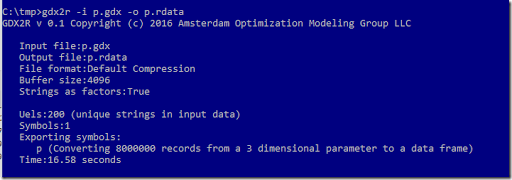Microsoft has acquired Revolution. Here are some early results of that.

#### Microsoft R Open

This is the standard R version with the addition of the Intel MKL library for high performance linear algebra (including multi-threading).

Also included is a neat way to make sure your users use the same versions of packages as you did. This will make reproducibility easier. Basically the idea is to add a checkpoint command with the snapshot date in your script that verifies that the same snapshot is used by your users. They call this the CRAN Time Machine.

The microsoft site is called mran.microsoft.com (MRAN = Microsoft R Application Network). The icon seems to be a monkey with glasses:

#### Microsoft R Server

This seems to be Linux only! I expected  a Windows port.

It has some out-of-core and parallel algorithms for very large data sets, and some connectivity to big-data databases. Looks like you can get this with your MSDN subscription

#### Microsoft SQL Server R Services

Here I recognize Microsoft again: R inside SQL Server.  Here in one sentence:

• Built-in advanced analytics– provide the scalability and performance benefits of building and running your advanced analytics algorithms directly in the core SQL Server transactional database

#### Microsoft Azure

This cloud-based thing Cloud Service Platform has also some R angle. See this tutorial.

## Sunday, February 7, 2016

### Experiments writing .Rdata files

While building a prototype .Rdata writer we developed a gdx2r application. This tool will convert a GAMS GDX file to an .RData file. Here we do some performance testing to get a feeling of how fast this is and how large the files are.

The first step is to generate a large, dense three dimensional parameter and export this to a GDX file. We can use compression to make the generated GDX file smaller at the expense of more CPU time.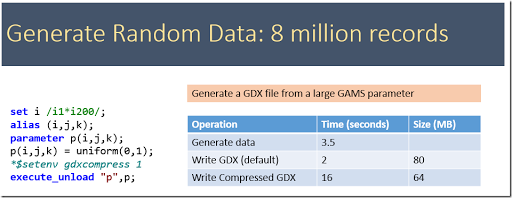To get some baseline data we first see how our gdx2sqlite tool behaves. A SQLite database is a convenient format to get data into R or Python. The –fast option helps very little here. This option typically has large impact on datasets with many smaller tables. The SQLite database is in general larger than a GDX file. A big advantage of using a database format is that we can make queries in R or Python to import suitable subsets of the data. In addition these queries can do some operations (summarizing, pivoting, transforming etc.).

A second much used format is CSV files. The GDXDUMP tool can generate this file. R has a read.csv command to import CSV files. The CSV format is limited to a single table.

Below we see that we can generate large SQLite files very fast: comparable to writing a CSV files. Typically we don’t get these speeds when doing inserts into a server based RDBMS (such as SQL Server or Oracle). In the tlimings below we used the uncompressed GDX file.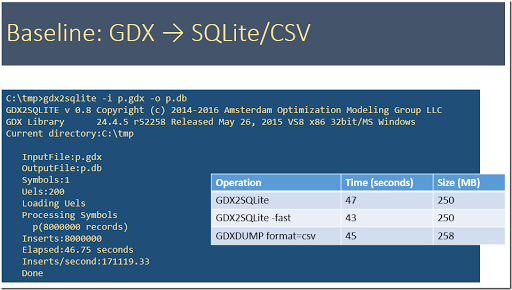Below are timings from the gdx2r tool. We notice that buffering before passing data to the next layer (either the file stream or the compressed file stream) really improves the speed. Even a small buffer of 1000 bytes is doing a good job (from 0 to 1k bytes makes more of a difference than from 1k to 10k bytes). The explanation for this is the following: the calls to the file stream are passed on to the Windows API WriteFile and these calls have a large fixed cost. A similar argument holds for compressed file stream. Because of the different building blocks (buffering vs no buffering, compression vs no compression) we have to try a number of possibilities.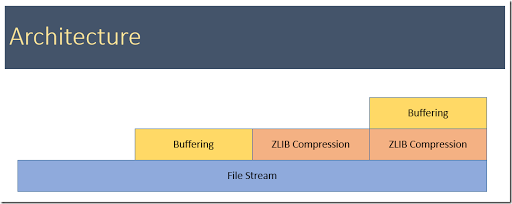In the table below, when bufsize=0, no buffering is used.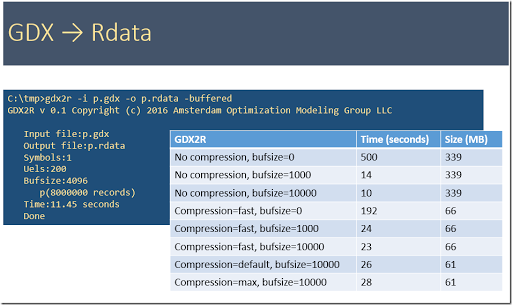Finally it is interesting to compare the reading times to get thigs into R. Below we have:

1. Read table p from the SQLite database. This is relatively fast.
2. Read the CSV file. The is very slow compared to the alternatives.
3. Load the .RData file is also fast.
4. The last entry is using the GAMS package gdxrrw. That package can read GDX files directly (assumes an installed GAMS system). This is somewhat slower than I would expect. It should be similar to SQLite and .Rdata timings but it is a factor of 2 slower.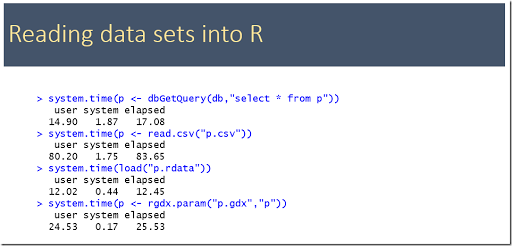## Saturday, February 6, 2016

### R: The RData File Format (2)

Now that we understand the .RData file format (as shown here) we can start writing some code to actually generate some .Rdata files. The tool gdx2r takes a GAMS GDX file and dumps the contents into an .Rdata file. E.g. in a GAMS model we can do:

execute "gdx2r -i results.gdx -o results.rdata"
;

The first command exports some GAMS symbols to a GDX file. The call to gdx2r converts it to an .Rdata file.

> setwd("c:/tmp")

i

f

c

x

> i

 "seattle"   "san-diego"

> f

 90

> c

i        j value

1   seattle new-york 0.225

2   seattle  chicago 0.153

3   seattle   topeka 0.162

4 san-diego new-york 0.225

5 san-diego  chicago 0.162

6 san-diego   topeka 0.126

> x

i        j level marginal lower upper

1   seattle new-york    50    0.000     0   Inf

2   seattle  chicago   300    0.000     0   Inf

3   seattle   topeka     0    0.036     0   Inf

4 san-diego new-york   275    0.000     0   Inf

5 san-diego  chicago     0    0.009     0   Inf

6 san-diego   topeka   275    0.000     0   Inf

>

Using the small result for the transportation model in the GAMS model library we show here a few symbols.

• i is one-dimensional set, so it is exported as a string vector.
• f is a scalar. In R these will arrive as a real vector of length one.
• c is a two-dimensional parameter. This will be represented as data frame. The i and j columns are represented as string vectors. I am thinking about changing this to a factor (may be using an option setting).  When using a factor we store a vector of unique strings (the levels) and then use an integer vector to index into that string vector. In R factors are often used to handle categorical variables.
• Finally x is a two-dimensional variable. This is also exported as a data frame.

The file results.rdata is a compressed binary file, so no good way to look at it directly: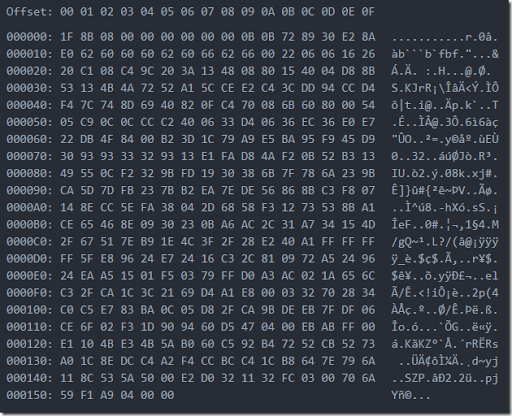For debugging I added some options to gdx2r. With the –ascii flag we can generate an ASCII version of this file. This looks like: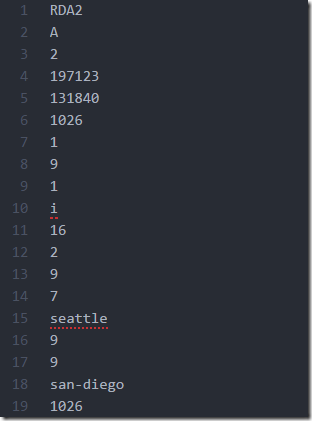We can also turn off compression using the flags –unbuffered or –buffered. That generates a binary file we can at least make some sense from:

All these versions can be read by R using the load command.

As we write these files natively (without going through R) we would expect this to be fast. Next post: some timings on large data.